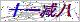••Product classification

Related article

# 西门子6RA70报警F007维修指定中心

## 6RA70专业维修西门子6RA70报警F007维修指定中心的详细资料：

`达到运行状态07后，经过P258的延时，输经济励磁电流P257. P257(F)= 0 (%P102) 停机励磁 6.限幅值参数设定P642.01-04= 100 % 主设定点速度限幅 P091=100% 斜坡给定阈值 P169=0 P170=0 带电流限幅的闭环电流控制 P605.01-04=1 转矩限幅 P171.01= 100% (P100为基值) ，P172.01=-100(P100为基值)电流限幅 7.斜坡函数发生器相关参数设定 P303.01(F)= 10 S P304.01(F)= 10S P305.01(F)= 0.5S P306.01(F) = 0.5S上述参数对斜坡参数组1进行设定规定了由0速到速的时间10S过度圆弧时间0.5S. 8.西门子6RA70报警F007维修指定中心辅助功能参数设定零速信号： P373(F)=1% 转速大于1%时状态字bit10 为1 P374(F)=0.5% 回环宽度 P375(F)=0.1S 延迟时间 P675(B) =24 CUD2板43端子开关量连接量24，当辅传动使用熔断器或超速开关时，接点动作产生外部故障1和2。 P689.1=B20 端子41，风机信号作为外部报警2 P771=106 设置开关量输出口1为装置故障状态输出 P755=167`

### 留言框

• #### 验证码：请输入计算结果（填写阿拉伯数字），如：三加四=7